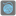• # Not making sense

## Question related to missionFlatten a List

So I solved this with a solution I found online: def flatlist(array): if array == []: return array if isinstance(array, list): return flatlist(array) + flatlist(array[1:]) return array[:1] + flatlist(array[1:])

But I originally had the following code which from my research should have worked, yet it would only append integers and not recursively call the function on a nested list. What am I missing?

```def flat_list(array):
flat = []
for a in array:
if type(a) is list:
flat_list(a)
else:
flat.append(a)
return flat
```